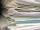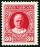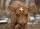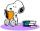# Algebra - math word problems

1. Iron collectingClass 7A collected 3.2 tonnes of iron more than class 7B. Together they collected 6.4 tonnes of iron to the secondary raw material collection. How much did each class collect?
2. Boys and girlsThere are 28 girls in the hall. 5/7 of all children are boys. How many children and how many boys are there?
3. Rate or interestAt what rate percent will Rs.2000 amount to Rs.2315.25 in 3 years at compound interest?
4. One hundred stampsA hundred letter stamps cost a hundred crowns. Its costs are four levels - twenty tenths , one crown, two-crown and five-crown. How many are each type of stamps? How many does the problem have solutions?The ladder is 10 m long The ladder is 8 m high How many meters is the distant heel from the wall?
6. Boys and girlsThere are 48 children in the sports club, boys are 10 more than girls. How many girls go to the club?
7. GroupsIn the 6th class there are 60 girls and 72 boys. We want to divide them into groups so that the number of girls and boys is the same. How many groups can you create? How many girls will be in the group?
8. Average ageThe average age of all people at the celebration was equal to the number of people present. After the departure of one person who was 29 years old, average age was again equal to the number present. How many people were originally to celebrate?
9. VacationTomas spent 60% of his savings for his weekly vacation. He was 32 € left. How many euros did he have before vacation?
10. ContainerThe container has a cylindrical shape the base diameter 0.8 m and the area of the base is equal to the area of the wall. How many liters of water can we pour into the container?
11. CalfThe weight of the calf meat obtained is 65% of the total weight of live calves. What was the weight of a calf from which 285 kg of meat was obtained?
12. Balance of accountTheo had a balance of -\$4 in his savings account. After making a deposit, he has \$25 in his account. What is the overall change to his account?
13. Trapezium internal anglesA trapezium where AB is parallel to CD, has angle A : angle D = 4 :5, angle B = 3x-15 and angle C = 4x+20. Find angle A, B, C and D.
14. Equation algebraogramSolve the equation: oco + ivo = cita How much has the task of solutions?
15. Alcohol mixtureThree liters of 96 percent alcohol we set up a certain amount of distilled water to form a 54-percent alcohol. How many liters of distilled water were used?
16. The bunsKate, Zofia and Peter Liked buns. Even today, their grandmother prepare their favorite meal. Katka eats 4 bunches, Žofia 3 and Petra eats 5 buns. Their grandmother said to them, "My inmate will you know how many buns I have been make today, if those you e
17. Gardens colonyGardens colony with dimensions of 180 m and 300 m are to be completely divided into the same large squares of the highest area. Calculate how many such squares can be obtained and determine the length of the square side.Calculate the volume and surface area of a regular quadrangular prism 35 cm high and the base diagonal of 22 cm.24 = n • 27, solve for nLMN is a right angled triangle with vertices at L(1,3), M(3,5) and N(6,n). Given angle LMN is 90° find n